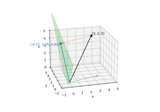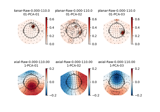# mne.compute_proj_raw¶

mne.compute_proj_raw(raw, start=0, stop=None, duration=1, n_grad=2, n_mag=2, n_eeg=0, reject=None, flat=None, n_jobs=1, meg='separate', verbose=None)[source]

Compute SSP (signal-space projection) vectors on continuous data.

This function aims to find those SSP vectors that will project out the `n` most prominent signals from the data for each specified sensor type. Consequently, if the provided input data contains high levels of noise, the produced SSP vectors can then be used to eliminate that noise from the data.

Parameters
rawinstance of `Raw`

A raw object to use the data from.

start`float`

Time (in sec) to start computing SSP.

stop`float`

Time (in sec) to stop computing SSP. None will go to the end of the file.

duration`float`

Duration (in sec) to chunk data into for SSP If duration is None, data will not be chunked.

n_grad`int`

Number of vectors for gradiometers.

n_mag`int`

Number of vectors for magnetometers.

n_eeg`int`

Number of vectors for EEG channels.

reject

Epoch rejection configuration (see Epochs).

flat

Epoch flat configuration (see Epochs).

n_jobs`int`

The number of jobs to run in parallel (default 1). Requires the joblib package. Number of jobs to use to compute covariance.

meg`str`

Can be ‘separate’ (default) or ‘combined’ to compute projectors for magnetometers and gradiometers separately or jointly. If ‘combined’, `n_mag == n_grad` is required and the number of projectors computed for MEG will be `n_mag`.

New in version 0.18.

verbose

If not None, override default verbose level (see `mne.verbose()` and Logging documentation for more). If used, it should be passed as a keyword-argument only.

Returns
projs: `list`

List of projection vectors.

## Examples using `mne.compute_proj_raw`¶